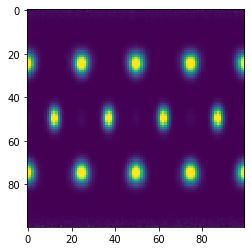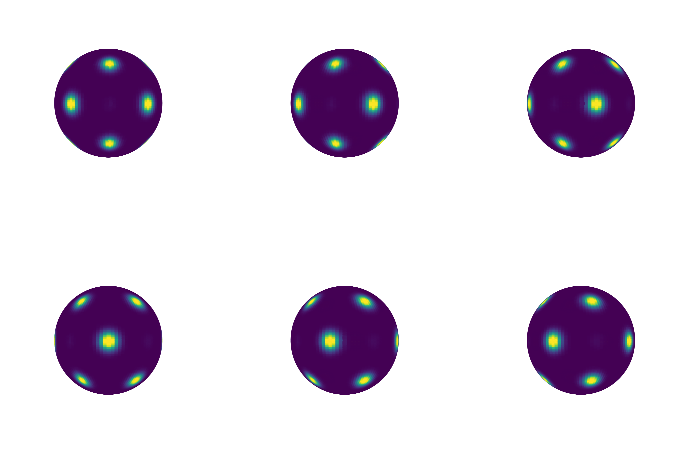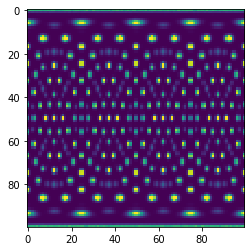# freud.environment.BondOrder#

## Computing Bond Order Diagrams#

The freud.environment module analyzes the local environments of particles. In this example, the freud.environment.BondOrder class is used to plot the bond order diagram (BOD) of a system of particles.

:

import freud
import matplotlib
import matplotlib.pyplot as plt
import numpy as np
from mpl_toolkits.mplot3d import Axes3D


### Setup#

Our sample data will be taken from an face-centered cubic (FCC) structure. The array of points is rather large, so that the plots are smooth. Smaller systems may need to gather data from multiple frames in order to smooth the resulting array’s statistics, by computing multiple times with reset=False.

:

uc = freud.data.UnitCell.fcc()
box, points = uc.generate_system(40, sigma_noise=0.05)


Now we create a BondOrder compute object and create some arrays useful for plotting.

:

n_bins_theta = 100
n_bins_phi = 100
bod = freud.environment.BondOrder((n_bins_theta, n_bins_phi))

phi = np.linspace(0, np.pi, n_bins_phi)
theta = np.linspace(0, 2 * np.pi, n_bins_theta)
phi, theta = np.meshgrid(phi, theta)
x = np.sin(phi) * np.cos(theta)
y = np.sin(phi) * np.sin(theta)
z = np.cos(phi)


### Computing the Bond Order Diagram#

Next, we use the compute method and the bond_order property to return the array.

:

bod_array = bod.compute(
system=(box, points), neighbors={"num_neighbors": 12}
).bond_order
# Clean up polar bins for plotting
bod_array = np.clip(bod_array, 0, np.percentile(bod_array, 99))
plt.imshow(bod_array.T)
plt.show()### Plotting on a sphere#

This code shows the bond order diagram on a sphere as the sphere is rotated. The code takes a few seconds to run, so be patient.

:

fig = plt.figure(figsize=(12, 8))
for plot_num in range(6):
ax = fig.add_subplot(231 + plot_num, projection="3d")
ax.plot_surface(
x,
y,
z,
rstride=1,
cstride=1,
facecolors=matplotlib.cm.viridis(bod_array / np.max(bod_array)),
)
ax.set_xlim(-1, 1)
ax.set_ylim(-1, 1)
ax.set_zlim(-1, 1)
ax.set_axis_off()
# View angles in degrees
view_angle = 0, plot_num * 15
ax.view_init(*view_angle)
plt.show()### Using Custom Neighbors#

We can also use a custom neighbor query to determine bonds. For example, we can filter for a range of bond lengths. Below, we only consider neighbors between $$r_{min} = 2.5$$ and $$r_{max} = 3$$ and plot the resulting bond order diagram.

:

bod_array = bod.compute(
system=(box, points), neighbors={"r_max": 3.0, "r_min": 2.5}
).bond_order
# Clean up polar bins for plotting
bod_array = np.clip(bod_array, 0, np.percentile(bod_array, 99))
plt.imshow(bod_array.T)
plt.show()